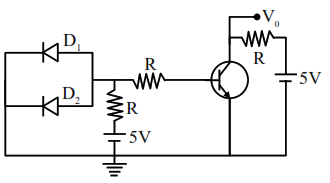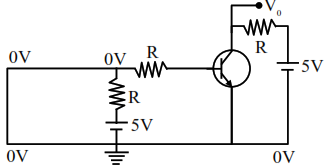# A circuit is arranged`
Question:

A circuit is arranged as shown in figure. The output voltage $V_{0}$ is equal to ....... V.Solution:

As diodes $D_{1}$ and $D_{2}$ are in forward bias, so they acted as neligible resistances

$\Rightarrow$ Input voltage become zero$\Rightarrow$ Input current is zero

$\Rightarrow$ Output current is zero

$\Rightarrow \mathrm{V}_{0}=5$ volt